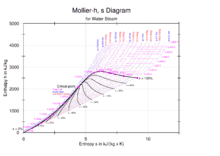# How do I find an enthalpy value if my temp. is below 50 deg. celcius?

I wanna design a curding machine (Milk to Cheese) with an open system that operate at isobaric process (contant pressure),
P = 1 atm = 1,... bar
the range of temperature is = 25-75 0C
can i find an enthalpy of water from Mollier Diagram if my temp. is 25 to 75 degree celcius ? how ?

from my view, i must seperates water properties from milk to make curds.
so i use mollier diagram but still confuse about it.#### Attachments

Chestermiller
Mentor
What is the heat capacity of water?

•bakharuddin
What is the heat capacity of water?
the amount of heat needed to raise its temperature a certain amount. One of water's most significant properties is that it takes a lot of heat to it to make it get hot. Precisely, water has to absorb 4,184 Joules of heat for the temperature of one kilogram of water to increase 1 degree celsius (°C)

Chestermiller
Mentor
the amount of heat needed to raise its temperature a certain amount. One of water's most significant properties is that it takes a lot of heat to it to make it get hot. Precisely, water has to absorb 4,184 Joules of heat for the temperature of one kilogram of water to increase 1 degree celsius (°C)
So, why can't you use this to get the enthalpy of water at 50 C (consistent with the Mollier diagram reference state)?

•bakharuddin
So, why can't you use this to get the enthalpy of water at 50 C (consistent with the Mollier diagram reference state)?

is there any equation to help me maybe ? still confuse to use this diagramChestermiller
Mentor
is there any equation to help me maybe ? still confuse to use this diagramWhy are you using a Mollier diagram? Why aren't you using the Steam Tables? The change in enthalpy with respect to temperature is $$\Delta H=mC_p\Delta t$$assuming no change of phase.

•russ_watters
Why are you using a Mollier diagram? Why aren't you using the Steam Tables? The change in enthalpy with respect to temperature is $$\Delta H=mC_p\Delta t$$assuming no change of phase.

because from mollier diagram there is an x value which is a moisture of water parameter, and i think is a must factor to include.
am i wrong ? or is it more simple using steam table to get the enthalpy ?

Chestermiller
Mentor
because from mollier diagram there is an x value which is a moisture of water parameter, and i think is a must factor to include.
am i wrong ? or is it more simple using steam table to get the enthalpy ?
The moisture parameter gives the fraction that’s vapor, but, in your case, you have purely a liquid stream, right? It is, of course, simpler to use the steam tables(or even the equation), and it’s more accurate than reading off a graph. You seem to need to review thetmo to get the basic concepts down pat.

•bakharuddin
The moisture parameter gives the fraction that’s vapor, but, in your case, you have purely a liquid stream, right? It is, of course, simpler to use the steam tables(or even the equation), and it’s more accurate than reading off a graph. You seem to need to review thetmo to get the basic concepts down pat.

Okay ill try it using the steam table.
Thanks you sir, i really appreciate that. glad someone can help here•Chestermiller